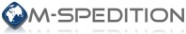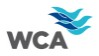Перейти к контенту

Главное меню:

Calculation of volume weight
At sending of cargoes by air we ask you to consider, that in all countries there is a well-known practice of calculation of volume weight of transported cargo which makes 167 kg for 1 cubic meter.
What is it and how it to count? The volume weight is the settlement size reflecting density of sent cargo since some departures have small physical weight, but they are volume enough. For their transportation the company will use the same resources, as for the heavy cargoes having the same volume, and the air vessel can contain only certain volume of cargo and no more.The formula is made for calculation of volume weight of a subject of type "box" under the formula:

Length (sm) x width (sm) x height (sm): 6000
Example: Cargo package dimensions (cm): length - 150, width - 100, height - 110.
Actual weight of a cargo package - 130 kg.
Calculation of volume weight according to the formula (cm): 150 x 100 x 110: 6000 = 275 kg.
At calculation of cost of the airfreight greatest of actual and volume scales is considered.
In this case calculation of cost of the airfreight is made at the rate of volume weight of cargo, since volume weight (275 kg) more than actual weight (130 kg).

The formula is made for calculation of volume weight of a subject of type "roll" under the following formula:
Volume weight in (kg) = (R*R*3.14*h)/6000
*Note: the Rounding off of fractional value of weight is made as a rule towards increase.
Rail wagon dimensions (PDF file)
Container dimensions (PDF file)